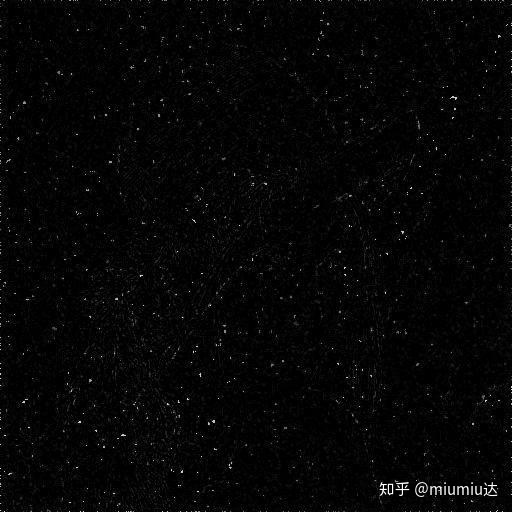• 本文摘自本人的毕业设计《数字图像滤波算法研究》2019, Chang’an University, Xi’an, China.本文适用于原始图像（即不含噪声...分别计算B、C的方差计算上述两项之比，得到SNR的值。对SNR的值对数化，得到以dB为...
本文摘自本人的毕业设计《数字图像滤波算法研究》2019, Chang’an University, Xi’an, China.本文适用于原始图像（即不含噪声的图像）存在的情况下。使用待计算SNR图像（记为A）与原始图像（记为B）做相减运算，得到一幅图像（记为C）；我们将B视为A的信息部分，将C视为A的噪声部分；分别计算B、C的方差；计算上述两项之比，得到SNR的值。对SNR的值对数化，得到以dB为单位的SNR值。附matlab代码clc,clear,close all  % 清理命令区、清理工作区、关闭显示图形warning off       % 消除警告feature jit off      % 加速代码运行A=imread(‘C:UsersLenovoDesktopdipxx.jpg’);B=imread(‘C:UsersLenovoDesktopdiporiginal.jpg’);C=imsubtract(A,B);avg1=mean2©;  %求图像均值[m,n]=size©;s1=0;for x=1:mfor y=1:ns1=s1+(C(x,y)-avg1)^2; %求得所有像素与均值的平方和。endendC1=s1/(mn); %利用方差公式求得avg2=mean2(B);  %求图像均值[m,n]=size(B);s2=0;for x=1:mfor y=1:ns2=s2+(B(x,y)-avg2)^2; %求得所有像素与均值的平方和。endendB1=s1/(mn); %利用方差公式求得D1=B1/C1;D1=10*log10(D1);example原始图像待检测SNR图像上面两图像相减得到的图像计算结果SNR=10.1861dB
展开全文• 在图像处理中经常会用到方差和局部方差的概念，这里就给出计算图像局部方法的代码，方便使用。 注：代码是关于导向滤波论文中的源码，大家可以自己下载 function dataVariance = getLocalVariance(srcData, ...
在图像处理中经常会用到方差和局部方差的概念，这里就给出计算图像局部方法的代码，方便使用。

注：代码是关于导向滤波论文中的源码，大家可以自己下载

function dataVariance = getLocalVariance(srcData, nHeight, nWidth, r)
% 计算局部方差
N               = boxfilter(ones(nHeight, nWidth), r);
srcDataMean     = boxfilter(srcData, r) ./ N;
srcDataDataMean = boxfilter(srcData.*srcData, r) ./ N;
dataVariance    = srcDataDataMean - srcDataMean .* srcDataMean;
end

function imDst = boxfilter(imSrc, r)

%   BOXFILTER   O(1) time box filtering using cumulative sum
%
%   - Definition imDst(x, y)=sum(sum(imSrc(x-r:x+r,y-r:y+r)));
%   - Running time independent of r;
%   - Equivalent to the function: colfilt(imSrc, [2*r+1, 2*r+1], 'sliding', @sum);
%   - But much faster.

[hei, wid] = size(imSrc);
imDst = zeros(size(imSrc));

%cumulative sum over Y axis
imCum = cumsum(imSrc, 1);
%difference over Y axis
imDst(1:r+1, :) = imCum(1+r:2*r+1, :);
imDst(r+2:hei-r, :) = imCum(2*r+2:hei, :) - imCum(1:hei-2*r-1, :);
imDst(hei-r+1:hei, :) = repmat(imCum(hei, :), [r, 1]) - imCum(hei-2*r:hei-r-1, :);

%cumulative sum over X axis
imCum = cumsum(imDst, 2);
%difference over Y axis
imDst(:, 1:r+1) = imCum(:, 1+r:2*r+1);
imDst(:, r+2:wid-r) = imCum(:, 2*r+2:wid) - imCum(:, 1:wid-2*r-1);
imDst(:, wid-r+1:wid) = repmat(imCum(:, wid), [1, r]) - imCum(:, wid-2*r:wid-r-1);
end




展开全文• 使用该.m文件可以计算二维图像的信息熵，平均灰度
• 采用矩阵移位相加与相减，避免了多层for循环，大大加快了程序的运行速度，而且4邻域与8邻域的权值可以调整
• 选择一幅灰度图像，用Matlab编程计算图像的灰度均值、方差和熵。如何插入一段漂亮的代码片 中的图片: 如何插入一段漂亮的代码片 // An highlighted block var foo = 'bar'; ...
原始图片:灰度均值：
% 求一副灰度图像的均值
close all;
clear;
clc;
i=imread('灰度.jpg'); %载入灰度图像
i=double(i);  %将uint8型转换为double型，否则不能计算统计量
% avg1=mean(i,1); %列向量均值
% avg2=mean(i,2); %行向量均值
% avg3=mean(i); %列向量均值
[m,n]=size(i);
s=0;
for x=1:m
for y=1:n
s=s+i(x,y); %求像素值总和 s
end
end
%所有像素均值
a=mean(mean(i))%先计算列向量均值，再求总均值。


结果：
a = 102.8351
方差：
% 求一副灰度图像的方差
close all
clear
clc;
i=imread('灰度.jpg'); %载入灰度图像
i=double(i);  %将uint8型转换为double型，否则不能计算统计量
% sq1=var(i,0,1); %列向量方差，第二个参数为0，表示方差公式分子下面是n-1,如果为1则是n
% sq2=var(i,0,2); %行向量方差
avg=mean2(i);  %求图像均值
[m,n]=size(i);
s=0;
for x=1:m
for y=1:n
s=s+(i(x,y)-avg)^2; %求得所有像素与均值的平方和。
end
end
%求图像的方差
a=var(i(:)) %利用函数var求得。

结果：a = 4.4803e+03
熵：
%求一幅图像的熵值
clc
clear
close all
I=imread('灰度.jpg');
[C,L]=size(I); %求图像的规格
Img_size=C*L; %图像像素点的总个数
G=256; %图像的灰度级
H_x=0;
nk=zeros(G,1);%产生一个G行1列的全零矩阵
for i=1:C
for j=1:L
Img_level=I(i,j)+1; %获取图像的灰度级
nk(Img_level)=nk(Img_level)+1; %统计每个灰度级像素的点数
end
end
for k=1:G  %循环
Ps(k)=nk(k)/Img_size; %计算每一个像素点的概率
if Ps(k)~=0; %如果像素点的概率不为零
H_x=-Ps(k)*log2(Ps(k))+H_x; %求熵值的公式
end
end
H_x  %显示熵值

结果：H_x = 7.3704


展开全文• 1. 求图像的均值close all;clear;clc;i=imread('d:/lena.jpg'); %载入真彩色图像路径i=... %将uint8型转换为double型，否则不能计算统计量[m,n]=size(i);s=0;for x=1:m for y=1:n s=s+i(x,y); %求像素值总和 s ，...
1. 求图像的均值close all;clear;clc;i=imread('d:/lena.jpg'); %载入真彩色图像路径i=rgb2gray(i); %转换为灰度图i=double(i);  %将uint8型转换为double型，否则不能计算统计量[m,n]=size(i);s=0;for x=1:m    for y=1:n        s=s+i(x,y); %求像素值总和 s  ， i(x,y)表示位于某个坐标下的像素值    endend%所有像素均值a1=mean(mean(i)); %第一种方法：先计算列向量均值，再求总均值。a2=mean2(i); %第二种方法：用函数mean2求总均值a3=s/(m*n);  %第三种方法：按公式计算，像素值总和除以像素个数。a4=sum(sum(i))/(m*n); %第四种方法：也是按公式计算，但是用sum来求像素值总和。2.求图像的标准差close allclearclc;i=imread('d:/lena.jpg'); %载入真彩色图像i=rgb2gray(i); %转换为灰度图i=double(i);  %将uint8型转换为double型，否则不能计算统计量avg=mean2(i);  %求图像均值[m,n]=size(i);s=0;for x=1:m    for y=1:n    s=s+(i(x,y)-avg)^2; %求得所有像素与均值的平方和。    endend%求图像的方差a1=var(i(:)); %第一种方法：利用函数var求得。a2=s/(m*n-1); %第二种方法：利用方差公式求得a3=(std2(i))^2; %第三种方法：利用std2求得标准差，再平方即为方差。
展开全文• 一、求均值% 求一副灰度图像的均值 close all; clear; clc; i=imread('d:/lena.jpg');... %将uint8型转换为double型，否则不能计算统计量 % avg1=mean(i,1); %列向量均值 % avg2=mean(i,2); %行向量均值 % avg3=mea
• 一。基本概念 图像相似度计算就是对...算法总结计算图像相似度的算法有很多，常见的有以下几种：1。基于直方图。直方图能够描述一幅图像中颜色的全局分布，是一种入门级的图像相似度计算方法。该算法计算过程很容...
• 本想再用滑动窗口计算写代码计算局部方差，网上查了下，matlab提供stdfilt函数来计算局部方差matlab内的解释是： stdfilt 图像的局部方差  语法  J = stdfilt(I)J = stdfilt(I, NHOOD) Description J = ...
• MATLAB图像基本运算1.均值函数代码函数调用2.中值函数代码函数调用3.众数函数代码函数调用4.方差函数代码函数调用5.协方差函数代码函数调用6.相关系数函数代码函数调用 1.均值 求出图像矩阵所有数值的均值。 注意点...
• Matlab计算热像图序列的平均值和方差1. 文件、数据介绍。2.运行程序，结果如下。3.源代码。 1. 文件、数据介绍。 文件夹下有55个格式为mat的热像图数据。 每个mat文件包含一个200*300的juzhen变量。 2.运行程序，...数学
• 二阶矩，反映待测区域颜色方差，即不均匀性 三阶矩，定义了颜色分量的偏斜度，即颜色的不对称性 close all;clear all;clc; J = imread('lena.jpg'); K = imadjust(J,[70/255 160/255],[]); figure; subplot(121),...
• 对比度明显的图片往往灰度分布均匀，常用图像灰度方差（标准差）进行衡量，标准差大则一般对比度明显，标准差小则一般对比度不明显。 下面讲解图像处理中常用的加强对比度的方法。1.对数变换对数变换为什么管用，...
• 二阶矩，反映待测区域颜色方差，即不均匀性 三阶矩，定义了颜色分量的偏斜度，即颜色的不对称性 close all;clear all;clc; J = imread('lena.jpg'); K = imadjust(J,[70/255 160/255],[]); figure; subplot...
• 该算法假定图像分为两类（符合双峰直方图分布，两类分别称为前景/目标像素和背景像素），然后计算出一个最优的阈值将将此图像分为两类使得其类间方差最大。Otsu是费舍尔离散判断分析的一维表现形式。 2 方法 二、...
• 该算法假定图像分为两类（符合双峰直方图分布，两类分别称为前景/目标像素和背景像素），然后计算出一个最优的阈值将将此图像分为两类使得其类间方差最大。Otsu是费舍尔离散判断分析的一维表现形式。 2 方法 二、...
• 该算法假定图像分为两类（符合双峰直方图分布，两类分别称为前景/目标像素和背景像素），然后计算出一个最优的阈值将将此图像分为两类使得其类间方差最大。Otsu是费舍尔离散判断分析的一维表现形式。 2 方法 二、...
• 课程设计任务书 学生姓名 专业班级 指导教师...采用MATLAB选用适当的函数或矩阵进行如下计算 极限的计算微分的计算积分的计算级数的计算求解代数方程求解 常微分方程 矩阵的最大值最小值均值方差转置逆行列式特征值的计
• 该算法假定图像分为两类（符合双峰直方图分布，两类分别称为前景/目标像素和背景像素），然后计算出一个最优的阈值将将此图像分为两类使得其类间方差最大。Otsu是费舍尔离散判断分析的一维表现形式。 2 方法 二、...
• 计算方差 计算灰阶频数 以上函数的测试脚本 测试脚本结果 寻找最大值和索引 function [MaxValue,iMaxValueIndex,jMaxValueIndex] = MaxValueFun(InputImage) %==========================================...
• 其余那些m std是算均值方差用的 跟计算熵没啥关系，不用自己就删掉 clear;close; [f,p] = uigetfile('*.*','选择图像文件'); I=imread(strcat(p,f)); %图片的均值与标准差计算 mr=mean(mean(I(:,:,1))); std_r=std2...
• ch3_3_1：计算图像的局部标准差（§3.3.1） ch3_3_2：计算输入图像的3×3邻域像素值的最大值（§3.3.2） ch3_4_1：根据指定的坐标选择一个六边形区域（§3.4.1） ch3_4_2：按灰度分割图像中的目标（§3.4.1） ch3_4_...
• 综合运用数字图像处理的理论知识对图像进行读入和输出图像图像格式转换、求直方图、滤波、去噪、图像的傅里叶变换等，再利用 MATLAB 作为编程工具进行计算机实现，从而加深对所学知识的理解，建立概念，提高动手...
• 因为MATLAB对于不同数据类型的图像，将方差归一化到了[0,1]之间，可以计算得到，uint8类型的图像，方差的范围为[0,255^2]（意思就是这种类型的图像方差最大只能达到255的平方，可以写出方差的计算公式看一下，当然这...
• 峰值信噪比PSNR~均方差MSE matlab实现 峰值信噪比：PSNR用来评价一幅图像压缩后和原图像相比质量的好坏。PSNR越高，压缩后失真越小，效果越好。均方差用MSE表示，均方差值越小越好。均方差是各数据偏离真实值的距离...计算机视觉# matlab计算图像方差matlab 订阅